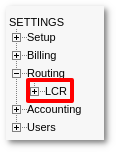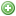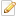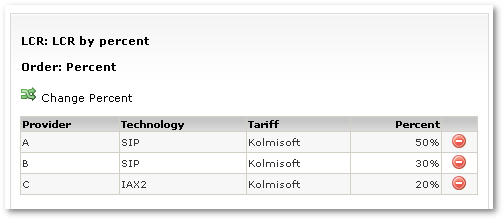# Routing by Percent

This type of routing selects a route by percentage value of Providers.

## Usage

To set this feature go to SETTINGS –> Billing –> LCRPressNew LCR to create new LCR or click onicon on existing LCR and set the order type of percent.

It is similar to Priority-based routing, except that here Providers are selected randomly with greater or lesser probability.## Example 1

For example, we have three providers with the following preferences:

```A - 50%
B - 30%
C - 20%
```

This means that:

• Provider A will be chosen FIRST with 50% probability.
• Provider B will be chosen FIRST with 30% probability.
• Provider C will be chosen FIRST with 20% probability.

MOR orders these providers using the following algorithm:

As a first step, it randomly selects the first provider. There is a 50% chance that Provider A will be selected, 30% for B, and 20% for C.

Lets say the randomly selected Provider is B.

At the second step, MOR selects from the remaining providers:

```A - 50%
C - 20%
```

There is a much greater chance that A will be randomly selected than C. So let's say A is selected.

The final order of our providers will be:

```B - A - C
```

Every time, the order is RANDOMLY done in the same way, using percent values for probability.

So we can get various results, such as A-B-C, A-C-B, B-A-C, C-A-B etc. BUT, following mathematical reasoning, when we make a huge amount of tries (going till infinity), sets will follow the rule in the first step: the Provider selected first will be A in 50% of the cases, B in 30%, and C in 20%.

## Example 2

To better illustrate the method, let's take a simpler situation. We have two providers with these percentages:

```A - 99%
B - 1%
```

Provider A will be selected first 99% of the time, so that when we have many ordered lists, they will look like:

```A-B, A-B, A-B, A-B, A-B, ........., B-A, A-B,........A-B, ..........
```

The probability that the provider order will be B-A is just 1%.

## Fail-over

This LCR ordering still keeps Fail-Over intact.

That is, after we have ordered the provider list, if the first provider in the list fails, the second will be dialed and so on.